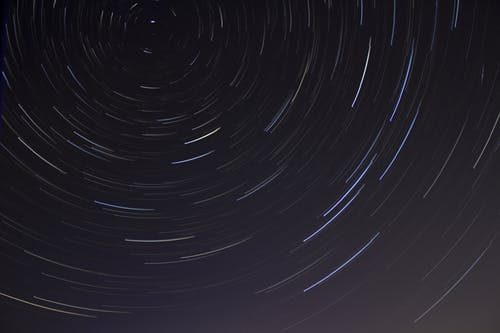Unit 8.4: "Plotting a Cosmi Collision Course": Force of Gravity in Solar System MotionsIn this unit, students use systems and system models ideas to explore the motions of the objects (elements) in the solar system. Students then learn the science concepts underlying the motions and describe how gravitational forces are involved when a large object (like the Sun) holds another much smaller object (like a planet or a human-made satellite) in its orbit. In exploring the forces controlling the system, students also determine how solar system elements are characterized and interact with each other, as well as how the patterns observed in our solar system seem to be prevalent in the greater universe.

Unit Challenge Question: How can we identify an unknown object traveling toward Earth and determine if it will collide with Earth?